#### FM442: Quantitative Methods for Finance and Risk Analysis

Jon Danielsson
Michaelmas Term 2021

We first load the packages for this seminar:

In :
library(rugarch)
library(tseries)
library(car)


## Univariate GARCH models¶

In this class we will work with the GARCH family, which was originally proposed in 1982 by Robert Engle. Most volatility models used in industry nowadays derive from this formulation.

Returns have a conditional distribution, which we will initially assume to be normal:

$$Y_{t} \sim \mathcal{N}\left(0,\sigma_t^2\right)$$

Which can be written as: $Y_t = \sigma_{t}Z_{t}$, where $Z_{t} \sim \mathcal{N}\left(0,1\right)$. $Z_t$ is called residual.

The $\textrm{GARCH}\left(L_1,L_2\right)$ model is:

$$\sigma_t^2 = \omega + \sum_{i=1}^{L_1}\alpha_{i}Y^2_{t-i} + \sum_{j=1}^{L_2}\beta_{j}\sigma^2_{t-j}$$

Where:
$\alpha$ is news.
$\beta$ is memory.
The size of $\left(\alpha + \beta\right)$ determines how quickly the predictability of the process dies out.

We are going to use the J.P. Morgan returns from our Y.RData file to run various GARCH type models discussed in Chapter 2.

In :
# Load the data

# Save the returns in a variable called y
y <- Y$JPM  In : # Simple plot plot(y, type = "l", main = "Returns for JP Morgan")In : # Autocorrelations source("acf_fm442.R") acf_fm442(y, main = "Autocorrelations of JPM returns") acf_fm442(y^2, main = "Autocorrelations of JPM returns squared")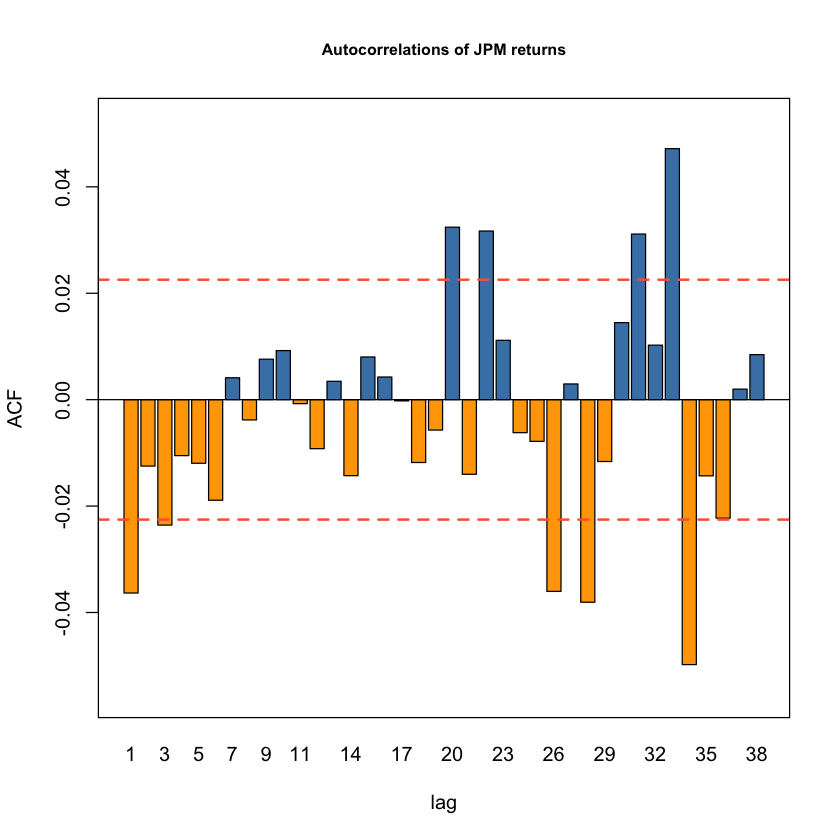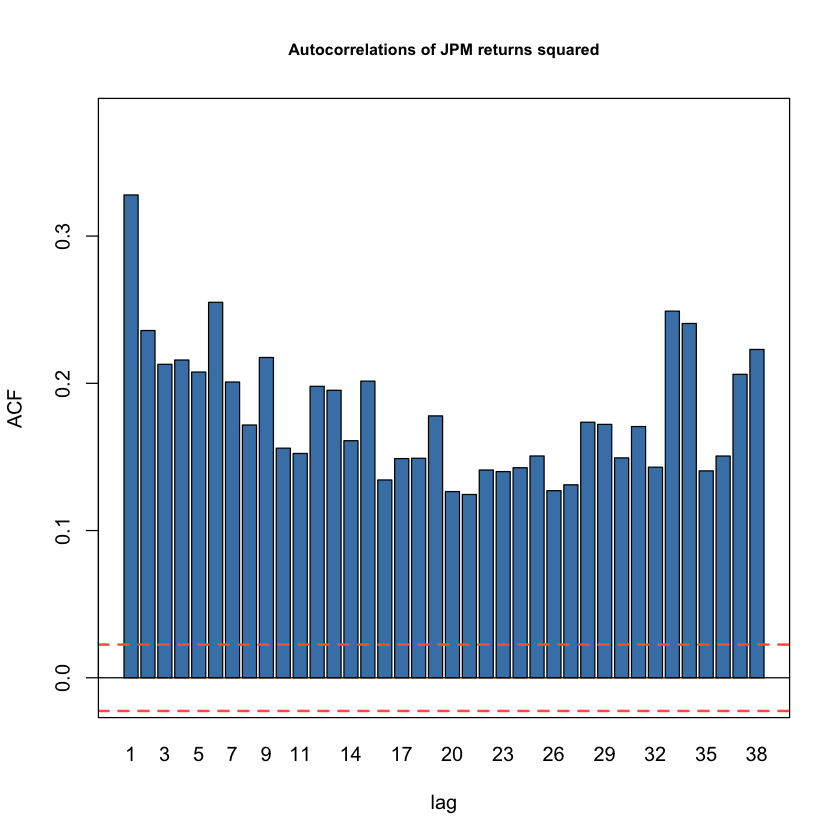The autocorrelation of the returns squared are significant for every lag, meaning that we see a behavior that can be modeled with GARCH. We will use the rugarch library for building univariate GARCH models. The documentation for it can be found here: https://cran.r-project.org/web/packages/rugarch/rugarch.pdf To build a univariate GARCH model, we first specify the type of GARCH model. ### GARCH model specification¶ We can specify the type of GARCH model we want to fit using the ugarchspec() function. For example, we can specify the number of lags, if the model includes leverage or power effects, whether the mean should be included or not, and the conditional distribution of the returns. The common syntax we will use inside ugarchspec() is: Type of model: variance.model = list(model = "type of model"), Whether to include or not a mean: mean.model = list(armaOrder = c(0,0), include.mean = FALSE), Type of conditiona distribution: distribution.model = "std" To get a detailed explanation on how to use all the options of ugarchspec(), you can type ?ugarchspec in the console. ### Default GARCH¶ First we will run the default version, which is a conditionally normal GARCH(1,1) model with non-zero mean which follows an ARMA(1,1) process: In : # Create the specifications default_spec <- ugarchspec()  In : # We can call the variable to see what's inside default_spec  *---------------------------------* * GARCH Model Spec * *---------------------------------* Conditional Variance Dynamics ------------------------------------ GARCH Model : sGARCH(1,1) Variance Targeting : FALSE Conditional Mean Dynamics ------------------------------------ Mean Model : ARFIMA(1,0,1) Include Mean : TRUE GARCH-in-Mean : FALSE Conditional Distribution ------------------------------------ Distribution : norm Includes Skew : FALSE Includes Shape : FALSE Includes Lambda : FALSE  Once we have specified the model, we can fit our data into it using ugarchfit(): In : # Fit the model to the data using ugarchfit default_garch <- ugarchfit(spec = default_spec, data = y)  In : # Call the variable default_garch  *---------------------------------* * GARCH Model Fit * *---------------------------------* Conditional Variance Dynamics ----------------------------------- GARCH Model : sGARCH(1,1) Mean Model : ARFIMA(1,0,1) Distribution : norm Optimal Parameters ------------------------------------ Estimate Std. Error t value Pr(>|t|) mu 0.000780 0.000129 6.0548 0.00000 ar1 0.986601 0.002568 384.2263 0.00000 ma1 -0.991540 0.000030 -33441.3465 0.00000 omega 0.000002 0.000002 1.3651 0.17223 alpha1 0.065173 0.011878 5.4869 0.00000 beta1 0.931240 0.012200 76.3326 0.00000 Robust Standard Errors: Estimate Std. Error t value Pr(>|t|) mu 0.000780 0.000866 0.90146 0.36734 ar1 0.986601 0.018888 52.23363 0.00000 ma1 -0.991540 0.000322 -3077.02735 0.00000 omega 0.000002 0.000014 0.16560 0.86847 alpha1 0.065173 0.093117 0.69991 0.48398 beta1 0.931240 0.096954 9.60499 0.00000 LogLikelihood : 19593.57 Information Criteria ------------------------------------ Akaike -5.1826 Bayes -5.1771 Shibata -5.1826 Hannan-Quinn -5.1807 Weighted Ljung-Box Test on Standardized Residuals ------------------------------------ statistic p-value Lag 3.123 0.07720 Lag[2*(p+q)+(p+q)-1] 4.512 0.01598 Lag[4*(p+q)+(p+q)-1] 5.518 0.34346 d.o.f=2 H0 : No serial correlation Weighted Ljung-Box Test on Standardized Squared Residuals ------------------------------------ statistic p-value Lag 8.272 0.004025 Lag[2*(p+q)+(p+q)-1] 12.901 0.001612 Lag[4*(p+q)+(p+q)-1] 14.839 0.003911 d.o.f=2 Weighted ARCH LM Tests ------------------------------------ Statistic Shape Scale P-Value ARCH Lag 1.313 0.500 2.000 0.2518 ARCH Lag 1.553 1.440 1.667 0.5784 ARCH Lag 2.707 2.315 1.543 0.5704 Nyblom stability test ------------------------------------ Joint Statistic: 46.3141 Individual Statistics: mu 0.1293 ar1 0.2486 ma1 0.2746 omega 7.3054 alpha1 0.3346 beta1 0.3797 Asymptotic Critical Values (10% 5% 1%) Joint Statistic: 1.49 1.68 2.12 Individual Statistic: 0.35 0.47 0.75 Sign Bias Test ------------------------------------ t-value prob sig Sign Bias 1.5290 1.263e-01 Negative Sign Bias 4.5360 5.822e-06 *** Positive Sign Bias 0.8293 4.070e-01 Joint Effect 22.8646 4.309e-05 *** Adjusted Pearson Goodness-of-Fit Test: ------------------------------------ group statistic p-value(g-1) 1 20 175.5 2.400e-27 2 30 172.4 2.485e-22 3 40 225.9 3.608e-28 4 50 257.0 5.509e-30 Elapsed time : 0.8550909  The function provides comprehensive information on the model fit. It includes the optimal parameters estimates, standard errors, tests for significance, likelihood, information criteria, and several other tests. Since this is too much information in the same place, we can tell R what particular information we are interested in. By using the @ symbol we can extract the contents from an object. For example, by running: In : # Check the content of default_garch names(default_garch@fit)  1. 'hessian' 2. 'cvar' 3. 'var' 4. 'sigma' 5. 'condH' 6. 'z' 7. 'LLH' 8. 'log.likelihoods' 9. 'residuals' 10. 'coef' 11. 'robust.cvar' 12. 'A' 13. 'B' 14. 'scores' 15. 'se.coef' 16. 'tval' 17. 'matcoef' 18. 'robust.se.coef' 19. 'robust.tval' 20. 'robust.matcoef' 21. 'fitted.values' 22. 'convergence' 23. 'kappa' 24. 'persistence' 25. 'timer' 26. 'ipars' 27. 'solver' We see all the content provided by the ugarchfit() function. We can access any element of this list by using the $ sign:

In :
# Extract a particular element
default_garch@fit$coef  mu 0.000780212961914253 ar1 0.986601360022316 ma1 -0.991540352497257 omega 2.26529403052011e-06 alpha1 0.0651731991646795 beta1 0.931240106506331 Another way of accessing this information is: In : # Extracting coefficients with coef() coef(default_garch)  mu 0.000780212961914253 ar1 0.986601360022316 ma1 -0.991540352497257 omega 2.26529403052011e-06 alpha1 0.0651731991646795 beta1 0.931240106506331 Let's say we are particularly interesting in$\omega$. We can access it by: In : # Accessing omega using its index coef(default_garch)  omega: 2.26529403052011e-06 However, using the name instead of the index can prevent potential mistakes in our code (for example, if the package is updated and the list reordered): In : # Accessing omega using its name coef(default_garch)["omega"]  omega: 2.26529403052011e-06 We can view the likelihood of the fitted model with: In : likelihood(default_garch)  19593.566174729 Since we are mostly interested in the coefficients and the likelihood of a fitted GARCH model, we are going to use a function that only outputs this information instead of calling the object and getting the other non-essential details. This function is called garch_fm442() and is inside the garch_fm442.R file: In : # Importing function that only outputs what we are interested in source("garch_fm442.R") # Call it garch_fm442(default_garch)  *---------------------------------* * GARCH Model Fit * *---------------------------------* Conditional Variance Dynamics ----------------------------------- GARCH Model : sGARCH(1,1) Mean Model : ARFIMA(1,0,1) Distribution : norm Optimal Parameters with Robust S.E.: ------------------------------------ Estimate Std. Error t value Pr(>|t|) mu 0.000780 0.000866 0.90146 0.36734 ar1 0.986601 0.018888 52.23363 0.00000 ma1 -0.991540 0.000322 -3077.02735 0.00000 omega 0.000002 0.000014 0.16560 0.86847 alpha1 0.065173 0.093117 0.69991 0.48398 beta1 0.931240 0.096954 9.60499 0.00000 LogLikelihood : 19593.57  ## Plotting the GARCH output¶ A very useful feature of rugarch package is plotting. If we call the function plot() on a fitted GARCH model like default_garch, the following interactive menu will appear: Make a plot selection (or 0 to exit): 1. Series with 2 Conditional SD Superimposed 2. Series with 1% VaR Limits 3. Conditional SD (vs |returns|) 4. ACF of Observations 5. ACF of Squared Observations 6. ACF of Absolute Observations 7. Cross Correlation 8. Empirical Density of Standardized Residuals 9. QQ-Plot of Standardized Residuals 10. ACF of Standardized Residuals 11. ACF of Squared Standardized Residuals 12. News-Impact Curve  We can visualize any of the plots by selecting the corresponding number. The plots for the default_garch are: 123456789101112These plots provide us with plenty of information to analyze the fit and results of our model. For example, from Plot 8 and Plot 9 we can see that the distribution of residuals in our model does not correctly fit under a normal distribution. As expected, there is an excess of observations around the mean in the histogram in Plot 8, and in Plot 9 we see the presence of fat tails in our observations. A key output of the GARCH model is the estimated conditional volatities, the sequence$\sigma_t$. This sequence is used for generating the first three plots shown above. We can access the sequence through default_garch@fit$sigma.

In :
# Plotting the estimated conditional volatility
lines(non_stationary_GARCH@fit$sigma, col="red") legend("topleft", legend = c("Stationary", "Non-stationary"), lty = 1, col = c("black", "red"))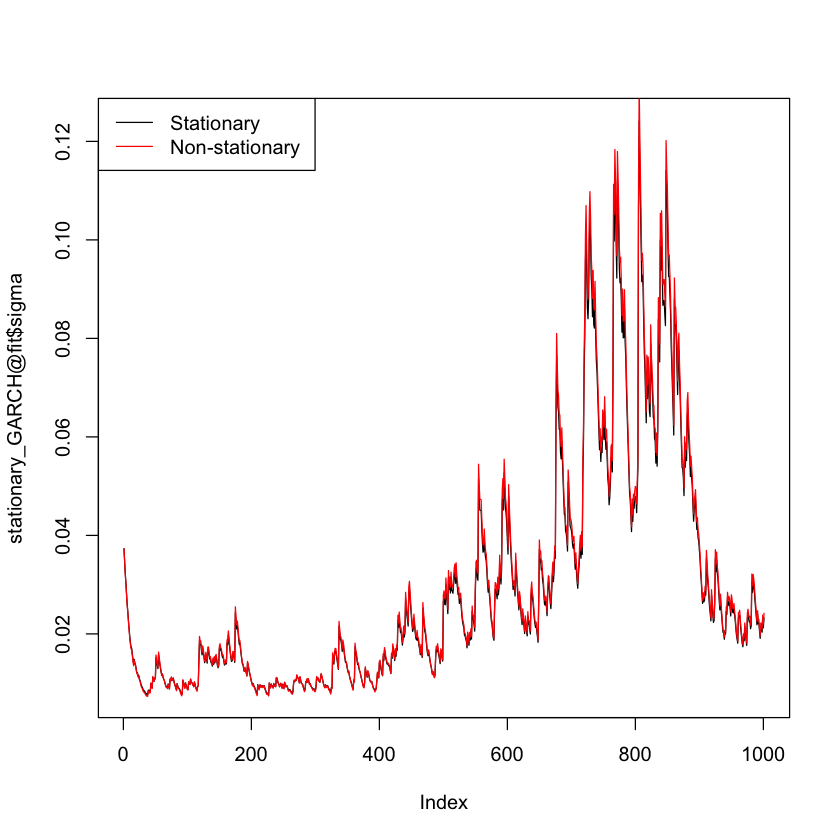However, they are not exactly the same: In : # Create a new variable with the absolute difference between stationary and non-stationary dif <- abs(stationary_GARCH@fit$sigma - non_stationary_GARCH@fit$sigma) # Plot it plot(dif, type = "l", main = "Distance in Stationary and Non-stationary models cond volatility")The distance between the estimated conditional volatility of the stationary and non-stationary model resembles the shape of the estimated conditional volatility. ## Assess Models and Testing¶ ### Summary of our models¶ The following table summarizes the coefficients for the models we have built, including the log-likelihood: ARCH GARCH tGARCH apARCH apARCH$\omega$0.00031 2.20394e-06 2.02425e-06 6.33423e-05 6.82302e-05$\alpha$0.47508 0.06364 0.07232 0.06583 0.07684$\beta$- 0.93288 0.92649 0.93867 0.93212$\nu$- - 6.04498 - 6.40025$\gamma$- - - 0.48650 0.48716$\delta$- - - 1.26031 1.22302$\textrm{Likelihood}$18408 19579 19787 19669 19859 ### Likelihood ratio test¶ Consider two models, where one is nested inside the other. This can be the case of the$\textrm{ARCH(1)}$, which is a subset of a$\textrm{GARCH(1,1)}$. We can call the nested model restricted (R), and the other unrestricted (U). By definition: $$\log \mathcal{L}_R \leq \mathcal{L}_U$$ Two times the difference between the unrestricted and restricted likelihood is distributed as a$\chi^2$with degrees of freedom equal to the number of restrictions: $$\textrm{LR} = 2\left(\mathcal{L}_U - \mathcal{L}_R\right) \sim \chi^2_{\left(\textrm{number of restrictions}\right)}$$ The number of restrictions means how many parameters we are excluding in the restricted model. For example, if we consider the ARCH vs GARCH models: In : # Number of restrictions ARCH vs GARCH coef(ARCH) coef(GARCH)  omega 0.000313176065702912 alpha1 0.47508527786574 omega 2.20394390496762e-06 alpha1 0.0636374926486844 beta1 0.932877579050624 The ARCH model, nested inside the GARCH model, has one parameter less. So in this case the number of restrictions which serves as the degrees of freedom in the chi-square distribution, would be one. In the case of the ARCH vs the tGARCH model: In : # Number of restrictions ARCH vs tGARCH coef(ARCH) coef(tGARCH)  omega 0.000313176065702912 alpha1 0.47508527786574 omega 2.02425289596277e-06 alpha1 0.0723187045621269 beta1 0.926491969979386 shape 6.04498046694411 The ARCH model has two parameters while the tGARCH has four, so we would be dealing with two degrees of freedom. We can easily implement the LR test using the likelihood output from the ugarchfit() function. Let's perform the test for the ARCH(1) and GARCH(1,1) models we created: In : # Creating the LR statistic - using cat() for output display cat(" Likelihood of ARCH: ", round(likelihood(ARCH),2), "\n", "Likelihood of GARCH: ", round(likelihood(GARCH),2), "\n", "2 * (Lu - Lr): ", round(2*(likelihood(GARCH)-likelihood(ARCH)),2))   Likelihood of ARCH: 18408.31 Likelihood of GARCH: 19578.86 2 * (Lu - Lr): 2341.11 The value of the test statistic is 2341. How do we know if this is above the critical value for a Chi-square with one degree of freedom and a confidence level of 95%? We can use the qchisq() function to find the 95% quantile of the Chi-square. In : # 95% quantile of chi-square qchisq(p = 0.95, df = 1)  3.84145882069412 The critical value is 3.84, which is smaller than 2341. This means we have enough evidence to reject that the two models perform similarly. We can also use pchisq() to find the p-value of the test statistic: In : # Adding the p-value p.value <- 1 - pchisq(2*(likelihood(GARCH)-likelihood(ARCH)), df = 1) cat(" Likelihood of ARCH: ", round(likelihood(ARCH),2), "\n", "Likelihood of GARCH: ", round(likelihood(GARCH),2), "\n", "2 * (Lu - Lr): ", round(2*(likelihood(GARCH)-likelihood(ARCH)),2), "\n", "p-value:", p.value)   Likelihood of ARCH: 18408.31 Likelihood of GARCH: 19578.86 2 * (Lu - Lr): 2341.11 p-value: 0 We can create a Test() function that takes two fitted models and gives an output with the likelihoods and p-values: In : # Creating a function for Likelihood Tests Test <- function(restricted, unrestricted) { # Specifying the degrees of freedom as the number of restrictions df <- length(unrestricted@fit$coef) - length(restricted@fit$coef) # Creating the statistic lr <- 2*(likelihood(unrestricted) - likelihood(restricted)) # Finding its p-value p.value <- 1 - pchisq(lr, df) # Output cat("Degrees of freedom:", df, "\n", "Likelihood of unrestricted model:", likelihood(unrestricted), "\n", "Likelihood of restricted model:", likelihood(restricted), "\n", "LR: 2*(Lu-Lr):", lr, "\n", "p-value:", p.value ) }  Using our new Test() function, let's test the GARCH and tapARCH models: In : # Testing the GARCH and tapARCH Test(GARCH, tapARCH)  Degrees of freedom: 3 Likelihood of unrestricted model: 19858.52 Likelihood of restricted model: 19578.86 LR: 2*(Lu-Lr): 559.3062 p-value: 0 ### Residual Analysis¶ We have assumed returns to be conditionally normally distributed. We can test this with a Jarque-Bera test for normality and a Ljung-Box test for autocorrelation of returns and returns-squared, and do a QQ plot to see the fit. Let's perform this test with the output of our GARCH(1,1) model: In : # Performing residual analysis residuals <- GARCH@fit$residuals

In :
# Autocorrelation of residuals
acf_fm442(residuals, main = "Autocorrelation of residuals")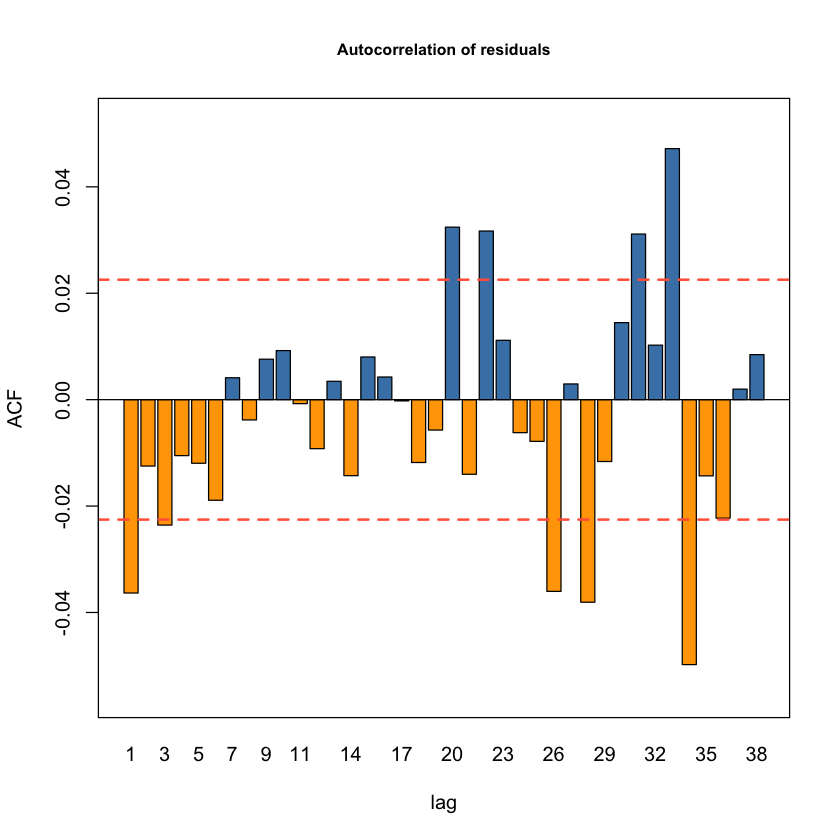In :
# Autocorrelation of residuals squared
acf_fm442(residuals^2, main = "Autocorrelation of residuals squared")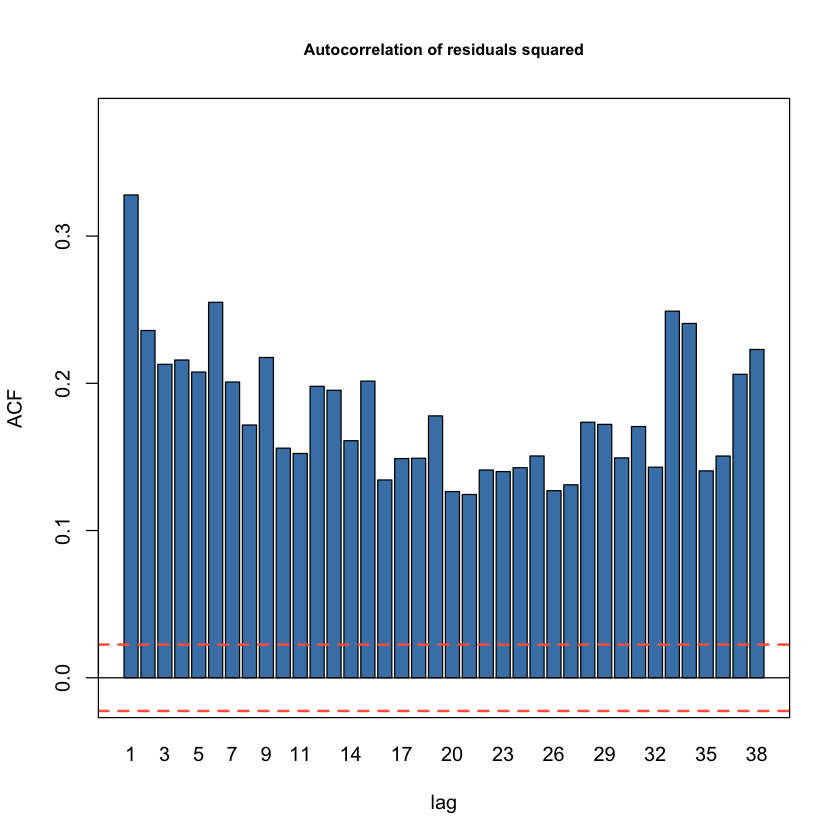In :
# Jarque-Bera test for Normality
jarque.bera.test(residuals)

	Jarque Bera Test

data:  residuals
X-squared = 43342, df = 2, p-value < 2.2e-16

In :
# Ljung-Box test for residuals
Box.test(residuals, type = "Ljung-Box")

	Box-Ljung test

data:  residuals
X-squared = 9.9875, df = 1, p-value = 0.001576

In :
# Ljung-Box test for residuals squared
Box.test(residuals^2, type = "Ljung-Box")

	Box-Ljung test

data:  residuals^2
X-squared = 813.18, df = 1, p-value < 2.2e-16

In :
# qqPlot for residuals under a normal distribution
qqPlot(residuals)

1. 4803
2. 4804